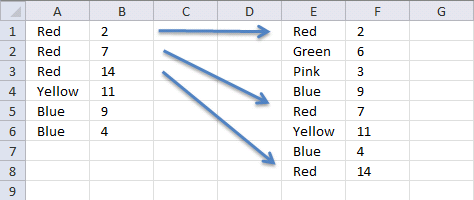Author: Oscar Cronquist Article last updated on December 30, 2018Linda asks in this post: How to return multiple values using vlookup in excel

I tried using the formula above but it didn't work for me and I can't figure out how to adjust it to accomodate my needs.

Here is what I have: Data Range is in \$E\$1:\$F\$8, I would like my results in Col. B. Lookup value in column A and return the value in Col F that matches.

Since there are duplicates in Col. A I want Col. B to return the next matching value from col. F.

Essentially this is a Vlookup with multiple matches that would return a different value. Thanks for any help you can provide.

Data Range Col. A Col B
Red 2 Red
Green 6 Red
Pink 3 Red
Blue 9 Yellow
Red 7 Blue
Yellow 11 Blue
Blue 4
Red 14

Array Formula in cell B1:

=INDEX(\$F\$1:\$F\$8, SMALL(IF(A1=\$E\$1:\$E\$8, ROW(\$E\$1:\$E\$8)-MIN(ROW(\$E\$1:\$E\$8))+1, ""), COUNTIF(A1:\$A\$1, A1)))

How to enter an array formula

1. Select cell B2
2. Type the formula above
3. Press and hold CTRL + SHIFT
4. Press Enter
5. Release all keys

If you did it right, the formula now has curly brackets before and after, like this: {=array_formula}.

Copy cell B1 and paste it down as far as needed.

### Explaining formula in cell B1

#### Step 1 - Find value

A1=\$E\$1:\$E\$8

becomes

="Red={"Red"; "Green"; "Pink"; "Blue"; "Red"; "Yellow"; "Blue"; "Red"}

and returns

{TRUE; FALSE; FALSE; FALSE; TRUE; FALSE; FALSE; TRUE}

#### Step 2 - Replace TRUE with corresponding row number

The IF function has three arguments, the first one must be a logical expression. If the expression evaluates to TRUE then one thing happens (argument 2) and if FALSE another thing happens (argument 3).

IF(A1=\$E\$1:\$E\$8, ROW(\$E\$1:\$E\$8)-MIN(ROW(\$E\$1:\$E\$8))+1, "")

becomes

IF({TRUE; FALSE; FALSE; FALSE; TRUE; FALSE; FALSE; TRUE}, ROW(\$E\$1:\$E\$8)-MIN(ROW(\$E\$1:\$E\$8))+1, "")

becomes

IF({TRUE; FALSE; FALSE; FALSE; TRUE; FALSE; FALSE; TRUE}, {1;2;3;4;5;6;7;8}, "")

and returns

{1;"";"";"";5;"";"";8}

#### Step 3 - Extract k-th smallest row number

To be able to return a new value in a cell each I use the SMALL function to filter row numbers from smallest to largest based on corresponding value.

SMALL(IF(A1=\$E\$1:\$E\$8, ROW(\$E\$1:\$E\$8)-MIN(ROW(\$E\$1:\$E\$8))+1, ""), COUNTIF(A1:\$A\$1, A1))

becomes

SMALL({1;"";"";"";5;"";"";8}, COUNTIF(A1:\$A\$1, A1))

The COUNTIF function counts values based on a condition or criteria, the first argument contains an expanding cell reference, it grows when the cell is copied to cells below. This lets the formula count values.

SMALL({1;"";"";"";5;"";"";8}, 1)

and returns 1.

#### Step 4 - Return value based on row number

The INDEX function returns a value based on a cell reference and column/row numbers.

INDEX(\$F\$1:\$F\$8, SMALL(IF(A1=\$E\$1:\$E\$8, ROW(\$E\$1:\$E\$8)-MIN(ROW(\$E\$1:\$E\$8))+1, ""), COUNTIF(A1:\$A\$1, A1)))

becomes

INDEX(\$F\$1:\$F\$8, 1)

and returns 2 in cell B1.